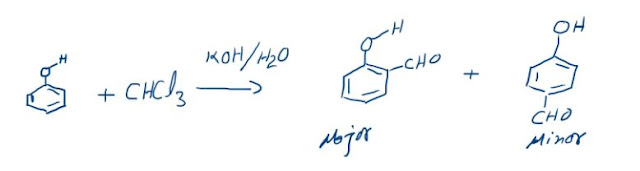## Bohrs Atomic Model

Bohr based his model on the observations of atomic spectrum of hydrogen. He gave the following postulates:
1. Electrons revolve around the nucleus in circular orbits also called “Energy Levels” or “Shells”. As long as an electron is revolving in an orbit it neither loses nor gains energy. Hence these orbits are called stationary states. Each stationary state is associated with a definite amount of energy and it is also known as energy levels. The greater the distance of the energy level from the nucleus, the more is the energy associated with it. The different energy levels are numbered as 1,2,3,4, (from nucleus onwards) or K, L, M, N etc.
2. Energy of the shells is fixed for a given atom that is an electron does not lose or gain energy when it’s present in the same shell.
3. When electron gains energy it gets excited to higher energy levels and when it gets de-excited it loses energy in form of electromagnetic radiations and comes to lower energy levels or ground state.

4. An electron revolves due to the centripetal force provided by the electrostatic attraction that is kq+q-/r2 from the nucleus. This centripetal force is balanced by a centrifugal force of circular motion i.e. mv2/r.
(k q+q-/r2) = (mv2/r)
5. The angular momentum of an electron in an orbit is an integral multiple of h/2p.
That is just like the energy of an electron the angular momentum is also quantized.
mvr = nh/2π

### Calculation of Radius and Energy Levels of Hydrogen Atom

The total energy, E of the electron is the sum of kinetic energy and potential energy.
Kinetic energy of the electron = ½ mv2
Potential energy = -KZe2 /r
Total energy = 1/2 mv2 – KZe2 /r
Or    En = - 1312/n2 x Z2 kJ /mole

### What does the negative electron energy (En) means?

The energy of the electron in a hydrogen atom has a –ve sign for all possible orbits.
This negative sign means that the energy of the electron in the atom is lower than the energy of a free electron at rest. An electron in an atom is because of attractive force due to protons in the nucleus. A free electron at rest in an atom that is infinitely far away from the nucleus and is assigned the energy value of zero. Mathematically, this corresponds to setting n equal to infinity in the equation so that Eα= 0. As electron gets closer to the nucleus, En becomes larger in absolute value and more and more negative. The most –ve energy value is given by n = 1 which corresponds to the most stable orbit.
ü As ‘n’ increases, we move away from the nucleus and thus radius increases.
ü As ‘Z’ increases, no. of protons increases, so forces of attraction increases and electron is pulled towards the nucleus, thus radius decreases.
ü As ‘n’ increases radius increases, therefore electrostatic force of attraction will decreases and thus centrifugal force will decrease and thus velocity will decrease.
Radius of the nth Bohr orbit
rn = 0.529 x n2/z Å
Merits of Bohr’s theory
a)   The experimental value of radii and energies in hydrogen atom are in good agreement with that calculated on the basis of Bohr’s theory.
b)  Bohr’s concept of stationary state of electron explains the emission and absorption spectra of hydrogen like atoms.
c)   The experimental values of the spectral lines of the hydrogen spectrum are in close agreement with that calculated by Bohr’s theory.
Limitations of Bohr’s theory
1.   It does not explain the spectra of atoms having more than one electron.
2.   Neils Bohr’s atomic model failed to account for the effect of magnetic field (Zeeman effect) or electric field (Stark effect) on the spectra of atoms or ions. It was observed that when the source of a spectrum is placed in a strong magnetic or electric field, each spectral line further splits into a number of lines. This observation could not be explained on the basis of Bohr’s model.

3.   De Broglie suggested that electrons like light have dual character. It has particle and wave character. Bohr treated the electron only as particle.
4.   Another objection to Bohr’s theory came from Heisenberg’s Uncertainty Principle. According to this principle “It is impossible to determine simultaneously the exact position and momentum of a small moving particle like an electron”.

### The Reimer-Tiemann reaction: Reaction and its Mechanism

Reimer-Tiemann Reaction Phenols on reaction with chloroform in the presence of sodium hydroxide (or potassium hydroxide) solution give hydro...